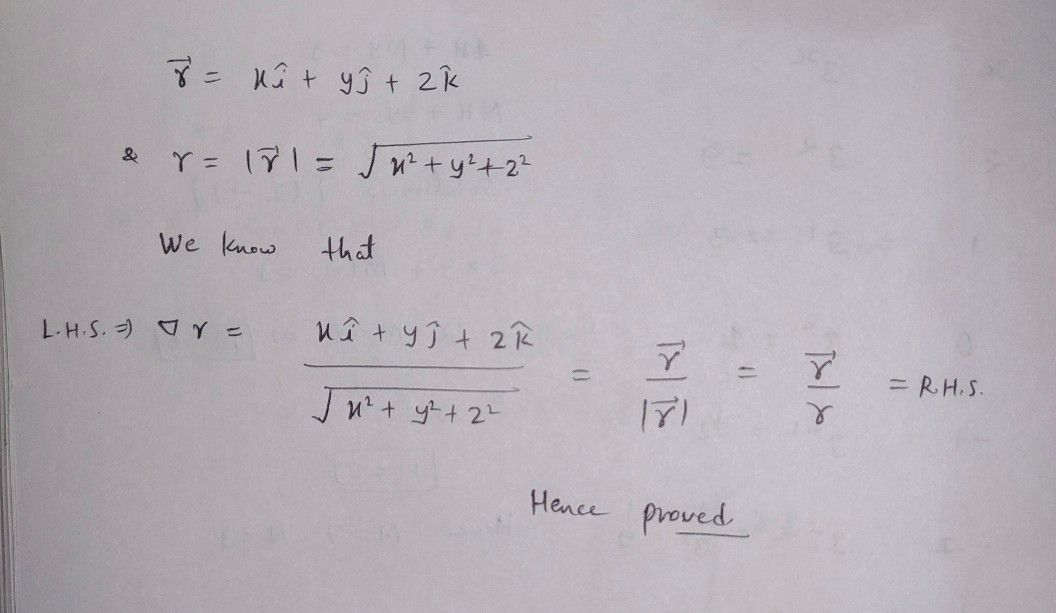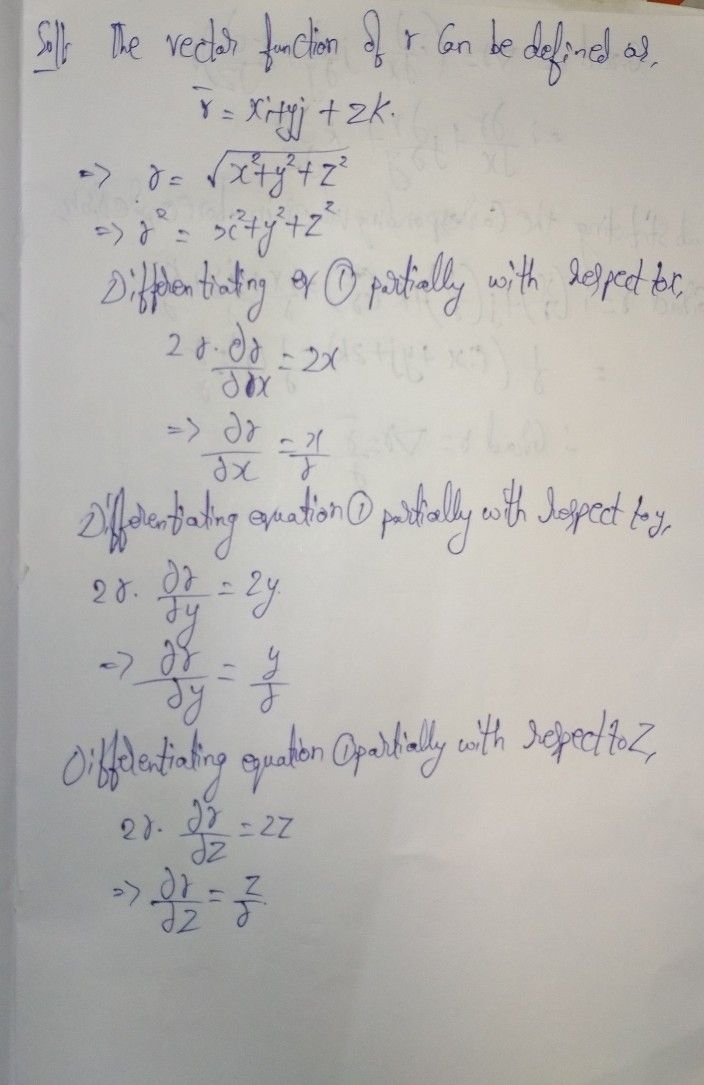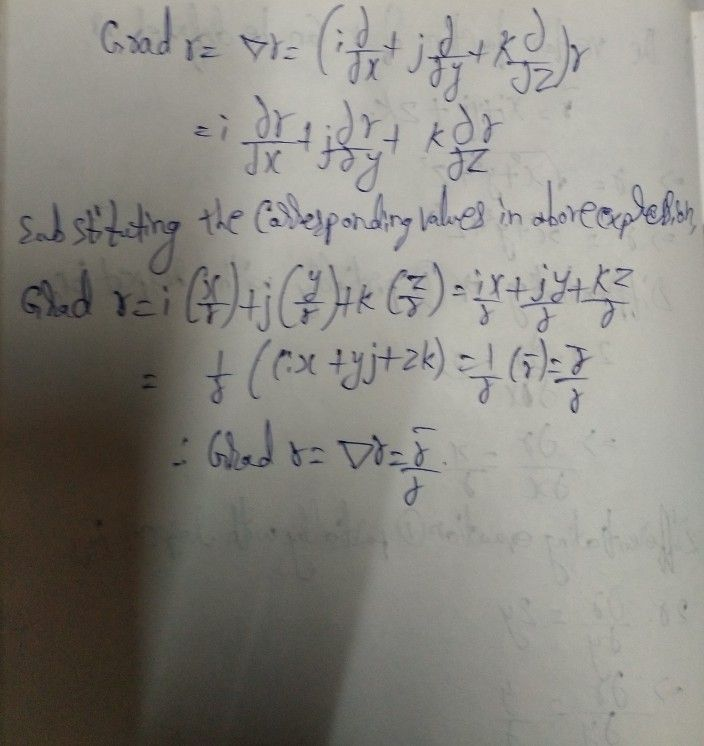Symbol
ProblemProve that $Vr=\dfrac {\vec{r} } {r}$ where $\vec{r} =x\vec{i} +y\vec{i} +$ zk and $r=1r1.$
Trigonometry
Search count: 107
SolutionQanda teacher - Availablehey dear feel free to ask queries
please evaluate the answer if satisfied
Please give 3000 coins as thank you gift this will not reduce your coins
happy Learning ??Student
very wrost answer and incorrectQanda teacher - AMUDHALAkindly check the answerStudent
thanks a much